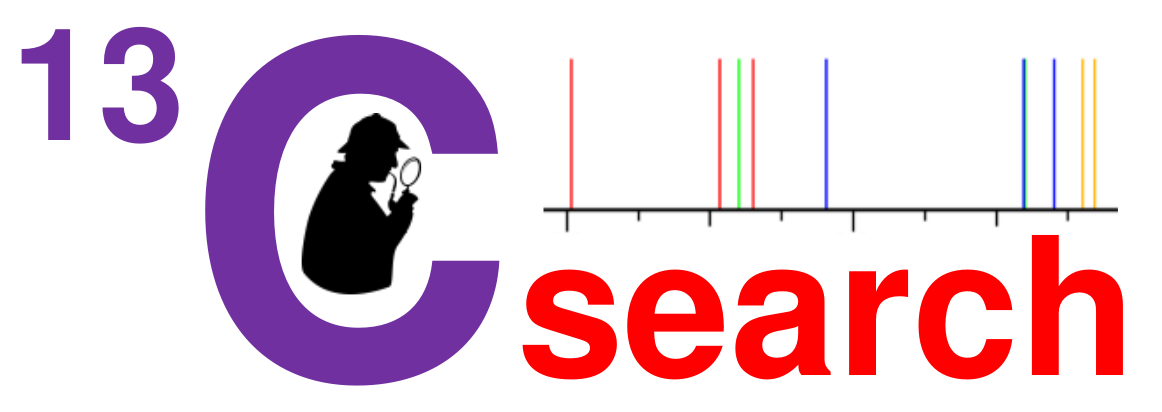## Spectral Similarity Search with RankingAccessing 615,365,172 predicted 13C-NMR spectraEnter CARBON chemical shift value (ppm) and MULTIPLICITY of your signals:

Allowed chemical shift range for 13C-signals is between -399.0 and +399.0 ppm

s = singulet ( Cquat ), d = doublet ( CH ), t = triplet ( CH2 ), q = quartet ( CH3 ),
e = even ( CH or CH3 ), o = odd ( Cquat or CH2 ), p = protonated ( CH or CH2 or CH3 ) , ? = unknown ( any type )

Couplings to other nuclei than protons (e.g. 31P, 19F) are ignored - enter only the shift values !
e.g. a CF3-group should be given as "122.8 s 2.5"

Deviation: 1.0 to 5.0 ppm allowed, useful values are somewhere around 3 ppm

Linenumber   Chemical Shift (ppm) Enter ALL LINES belonging to your unknown compound - entering only significant lines is insufficient In case your peaklist holds more than 20 lines then click here: 1 unknown s = C d = CH t = CH2 q = CH3 o = C,CH2 e = CH,CH3 p = CH,CH2,CH3 2 unknown s = C d = CH t = CH2 q = CH3 o = C,CH2 e = CH,CH3 p = CH,CH2,CH3 3 unknown s = C d = CH t = CH2 q = CH3 o = C,CH2 e = CH,CH3 p = CH,CH2,CH3 4 unknown s = C d = CH t = CH2 q = CH3 o = C,CH2 e = CH,CH3 p = CH,CH2,CH3 5 unknown s = C d = CH t = CH2 q = CH3 o = C,CH2 e = CH,CH3 p = CH,CH2,CH3 6 unknown s = C d = CH t = CH2 q = CH3 o = C,CH2 e = CH,CH3 p = CH,CH2,CH3 7 unknown s = C d = CH t = CH2 q = CH3 o = C,CH2 e = CH,CH3 p = CH,CH2,CH3 8 unknown s = C d = CH t = CH2 q = CH3 o = C,CH2 e = CH,CH3 p = CH,CH2,CH3 9 unknown s = C d = CH t = CH2 q = CH3 o = C,CH2 e = CH,CH3 p = CH,CH2,CH3 10 unknown s = C d = CH t = CH2 q = CH3 o = C,CH2 e = CH,CH3 p = CH,CH2,CH3 11 unknown s = C d = CH t = CH2 q = CH3 o = C,CH2 e = CH,CH3 p = CH,CH2,CH3 12 unknown s = C d = CH t = CH2 q = CH3 o = C,CH2 e = CH,CH3 p = CH,CH2,CH3 13 unknown s = C d = CH t = CH2 q = CH3 o = C,CH2 e = CH,CH3 p = CH,CH2,CH3 14 unknown s = C d = CH t = CH2 q = CH3 o = C,CH2 e = CH,CH3 p = CH,CH2,CH3 15 unknown s = C d = CH t = CH2 q = CH3 o = C,CH2 e = CH,CH3 p = CH,CH2,CH3 16 unknown s = C d = CH t = CH2 q = CH3 o = C,CH2 e = CH,CH3 p = CH,CH2,CH3 17 unknown s = C d = CH t = CH2 q = CH3 o = C,CH2 e = CH,CH3 p = CH,CH2,CH3 18 unknown s = C d = CH t = CH2 q = CH3 o = C,CH2 e = CH,CH3 p = CH,CH2,CH3 19 unknown s = C d = CH t = CH2 q = CH3 o = C,CH2 e = CH,CH3 p = CH,CH2,CH3 20 unknown s = C d = CH t = CH2 q = CH3 o = C,CH2 e = CH,CH3 p = CH,CH2,CH3 Click here when your peaklist holds more than 20 lines --->     <--- Click here when your peaklist holds more than 20 lines

 Global pattern of reference-spectrum: No other significant lines Other significant lines allowed

 Recommendation: Limit your result by molecular weight and number of signals in reference compounds Molecular weight from to Use constraint for display only      Use constraint already during search Number of signals from to
 Recommendation: Select presence / absence of elements in reference compounds F and P are easily seen from NMR, you might know presence / absence of N, Cl and Br from MS N O P S F Cl Br I other Element must be absent: Element may be absent or present: Element must be present:

Data must be given: Email for receiving result, projectname and name of peaklist/compound - select descriptive names

Email for receiving result:    *
Name of Project:    *
Name of Peaklist / Compound:    *